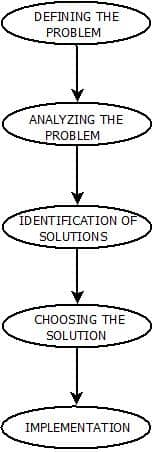# PROBLEM SOLVING IN AI

Problem Solving In Artificial Intelligence | Artificial Intelligence Tutorial

# Problem Solving In AI : Introduction

• Problem Solving in games such as “Sudoku” can be an example. It can be done by building an artificially intelligent system to solve that particular problem. To do this, one needs to define the problem statements first and then generating the solution  by keeping the conditions in mind.
• Some of the most popularly used problem solving with the help of artificial intelligence are:
1. Chess.
2. Travelling Salesman Problem.
3. Tower of Hanoi Problem.
4. Water-Jug Problem.
5. N-Queen Problem.

### Problem Searching

• In general, searching refers to as finding information one needs.
• Searching is the most commonly used technique of problem solving in artificial intelligence.
• The searching algorithm helps us to search for solution of particular problem.

### Problem

• Problems are the issues which comes across any system. A solution is needed to solve that particular problem.

### Steps : Solve Problem Using Artificial Intelligence

• The process of solving a problem consists of five steps. These are:Problem Solving in Artificial Intelligence
1. Defining The Problem: The definition of the problem must be included precisely. It should contain the possible initial as well as final situations  which should result in acceptable solution.
2. Analyzing The Problem: Analyzing the problem and its requirement must be done as few features can have immense impact on the resulting solution.
3. Identification Of Solutions: This phase generates reasonable amount of solutions to the given problem in a particular range.
4. Choosing a Solution: From all the identified solutions, the best solution is chosen basis on the results produced by respective solutions.
5. Implementation: After choosing the best solution, its implementation is done.